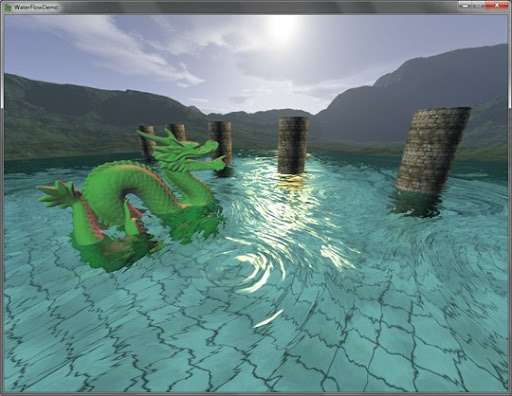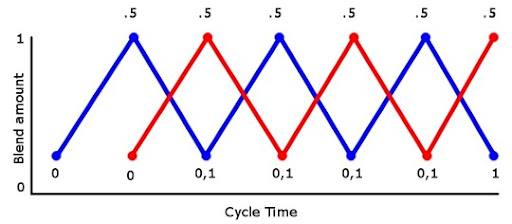## Tuesday, August 3, 2010

### Animating Water Using Flow MapsLast week I attended SIGGRAPH 2010, and among the many good presentations, Valve game a talk on the simple water shader they implemented for Left For Dead 2 and Portal 2. So on the plane ride back from LA, I whipped up this little sample from what I could remember of the talk. Edit: You can find the talk here: http://advances.realtimerendering.com/s2010/index.html

The standard technique for animated water is scrolling normal maps, as I’ve previously written about. The problem with this is that it looks unnatural as water does not uniformly move in one direction. So Valve came up with the idea of using flow maps ( based on a flow viz paper from the mid 90s ). The basic idea of flow maps is that you create a 2D texture that you will map to your water. And this map will contain the flow directions that you want the water to flow, with each pixel in the flow map representing a flow vector. This allows you to have varying velocity ( based on length of the flow vector ), and varying flow directions ( based on the color of the flow vector ). You then use this flow map to alter the texture coordinates of the normal maps instead of scrolling them. Lets get to work :)

The Flow Map

First we need to create a flow map. Here’s what I came up with in a couple of minutes in Photoshop. This flow map was designed around the column with dragon scene as with the previous scene. Note, this flow map is greatly exaggerated to demonstrate the effect.Using The Flow Map

Now we need to use the flow map to alter the water normal maps. We do this by taking the texture coordinate of the current water pixel and offset it using the flow vector from the flow map based on a time offset. We then render the water as we did in the previous water sample. But there’s a problem with this, after awhile the texture coordinates will become so distorted that the normal maps will be stretched and will have nasty filtering artifacts. So to solve this we limit the amount of distortion of the texture coordinates by resetting the time offset. This solves the over-distortion, but now the water will reset every X seconds. So we introduce another layer, that is offset from the first by half a time cycle. This will ensure that while one layer is fading out and beginning to reset, the next layer is fading to where the last layer was. Here’s a diagram to visualize this phase-in phase-out of the 2 layers.The graph illustrates that during a cycle time from 0 to 1, we want the layer to be fully interpolated at the mid-point in the cycle, and fully un-interpolated at 0 and 1. Lets see the code:
```//get and uncompress the flow vector for this pixel
float2 flowmap = tex2D( FlowMapS, tex0 ).rg * 2.0f - 1.0f;

float phase0 = FlowMapOffset0;
float phase1 = FlowMapOffset1;

// Sample normal map.
float3 normalT0 = tex2D(WaveMapS0, ( tex0 * TexScale ) + flowmap * phase0 );
float3 normalT1 = tex2D(WaveMapS1, ( tex0 * TexScale ) + flowmap * phase1 );

float flowLerp = ( abs( HalfCycle - FlowMapOffset0 ) / HalfCycle );
float3 offset = lerp( normalT0, normalT1, flowLerp );```
In the code above, HalfCycle would be .5 if our cycle was from 0 to 1. We can see here that we unwrap the flow vector (as it is stored in [0,1] and we need it in [-1,1]), fetch the normals using the flow vector and then lerp between the two normals based on the cycle time. This however will lead to a subtle pulsing affect, which I couldn’t really notice when the water was rendered, but I included the fix for completeness. To fix this pulsing effect, we perturb the flow cycle at each pixel using a noise map.
```//get and uncompress the flow vector for this pixel
float2 flowmap = tex2D( FlowMapS, tex0 ).rg * 2.0f - 1.0f;
float cycleOffset = tex2D( NoiseMapS, tex0 ).r;

float phase0 = cycleOffset * .5f + FlowMapOffset0;
float phase1 = cycleOffset * .5f + FlowMapOffset1;

// Sample normal map.
float3 normalT0 = tex2D(WaveMapS0, ( tex0 * TexScale ) + flowmap * phase0 );
float3 normalT1 = tex2D(WaveMapS1, ( tex0 * TexScale ) + flowmap * phase1 );

float flowLerp = ( abs( HalfCycle - FlowMapOffset0 ) / HalfCycle );
float3 offset = lerp( normalT0, normalT1, flowLerp );```
And that’s pretty much it. I’ll update the post/source when the slides are posted from SIGGRAPH in case I left anything out. Video time!

Source/Demo: WaterFlow Demo